# How to Calculate and Solve for Elongation Potential of Twin Deformation | Fracture Mechanics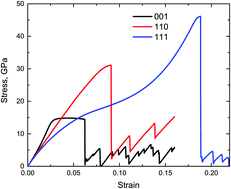The image above represents elongation potential of twin deformation.

To compute for elongation potential of twin deformation, two essential parameters are needed and these parameters are Distance between the 2 Slip Planes (S) and Angle of Deformation (x).

The formula for calculating elongation potential of twin deformation:

l’/l = (1 + Stanx)0.5

Where:

l’/l = Elongation Potential of Twin Deformation
S = Distance between the 2 Slip Planes
x = Angle of Deformation

Let’s solve an example;
Find the elongation potential of twin deformation when the distance between the 2 slip planes is 24 and the angle of deformation is 12°.

This implies that;

S = Distance between the 2 Slip Planes = 24
x = Angle of Deformation = 12°

l’/l = (1 + Stanx)0.5
l’/l = (1 + 24tan(12°))0.5
l’/l = (1 + 24(0.213))0.5
l’/l = (1 + 5.101)0.5
l’/l = (6.101)0.5
l’/l = 2.470

Therefore, the elongation potential of twin deformation is 2.470.

Nickzom Calculator – The Calculator Encyclopedia is capable of calculating the elongation potential of twin deformation.

To get the answer and workings of the elongation potential of twin deformation using the Nickzom Calculator – The Calculator Encyclopedia. First, you need to obtain the app.

You can get this app via any of these means:

To get access to the professional version via web, you need to register and subscribe for NGN 2,000 per annum to have utter access to all functionalities.
You can also try the demo version via https://www.nickzom.org/calculator

Android (Paid) – https://play.google.com/store/apps/details?id=org.nickzom.nickzomcalculator
Android (Free) – https://play.google.com/store/apps/details?id=com.nickzom.nickzomcalculator
Apple (Paid) – https://itunes.apple.com/us/app/nickzom-calculator/id1331162702?mt=8
Once, you have obtained the calculator encyclopedia app, proceed to the Calculator Map, then click on Materials and Metallurgical under Engineering.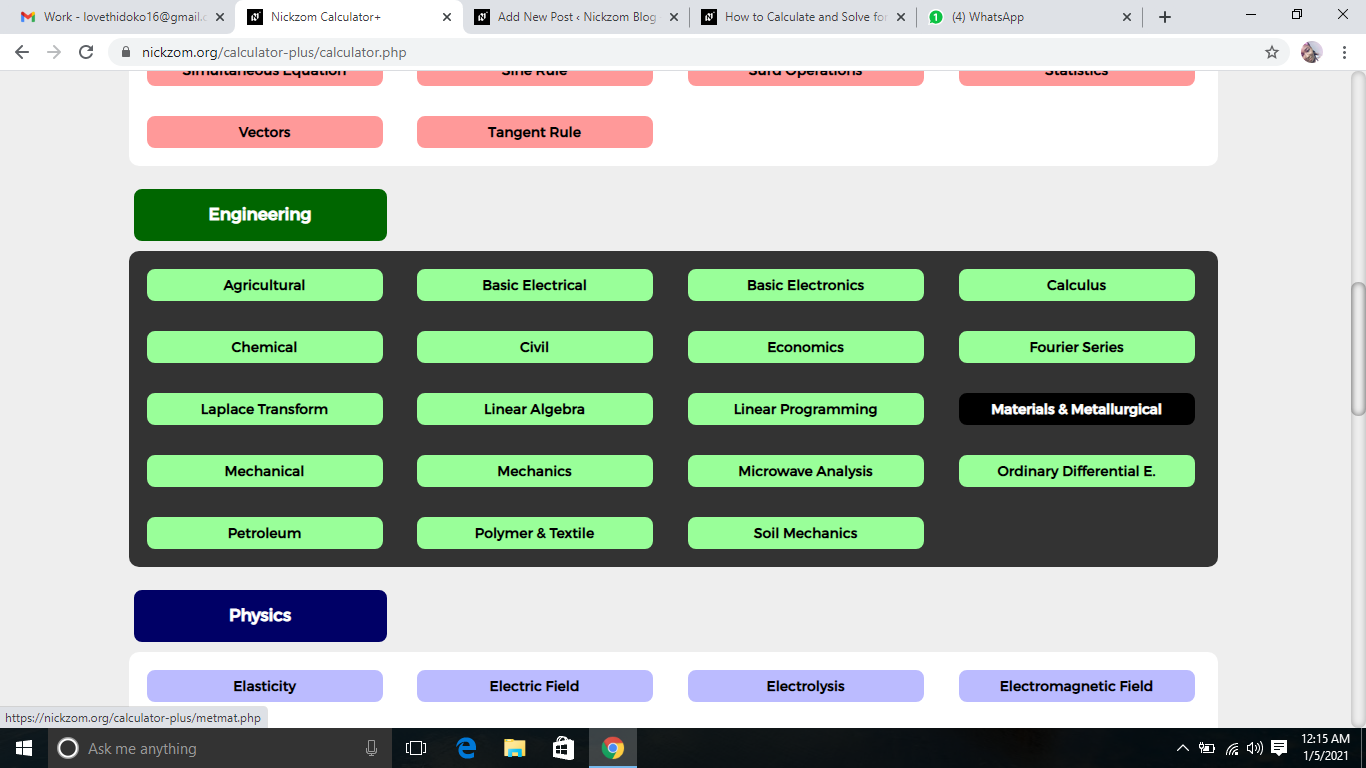Now, Click on Fracture Mechanics under Materials and MetallurgicalNow, Click on Elongation Potential of Twin Deformation under Fracture MechanicsThe screenshot below displays the page or activity to enter your values, to get the answer for the elongation potential of twin deformation according to the respective parameters which is the Distance between the 2 Slip Planes (S) and Angle of Deformation (x).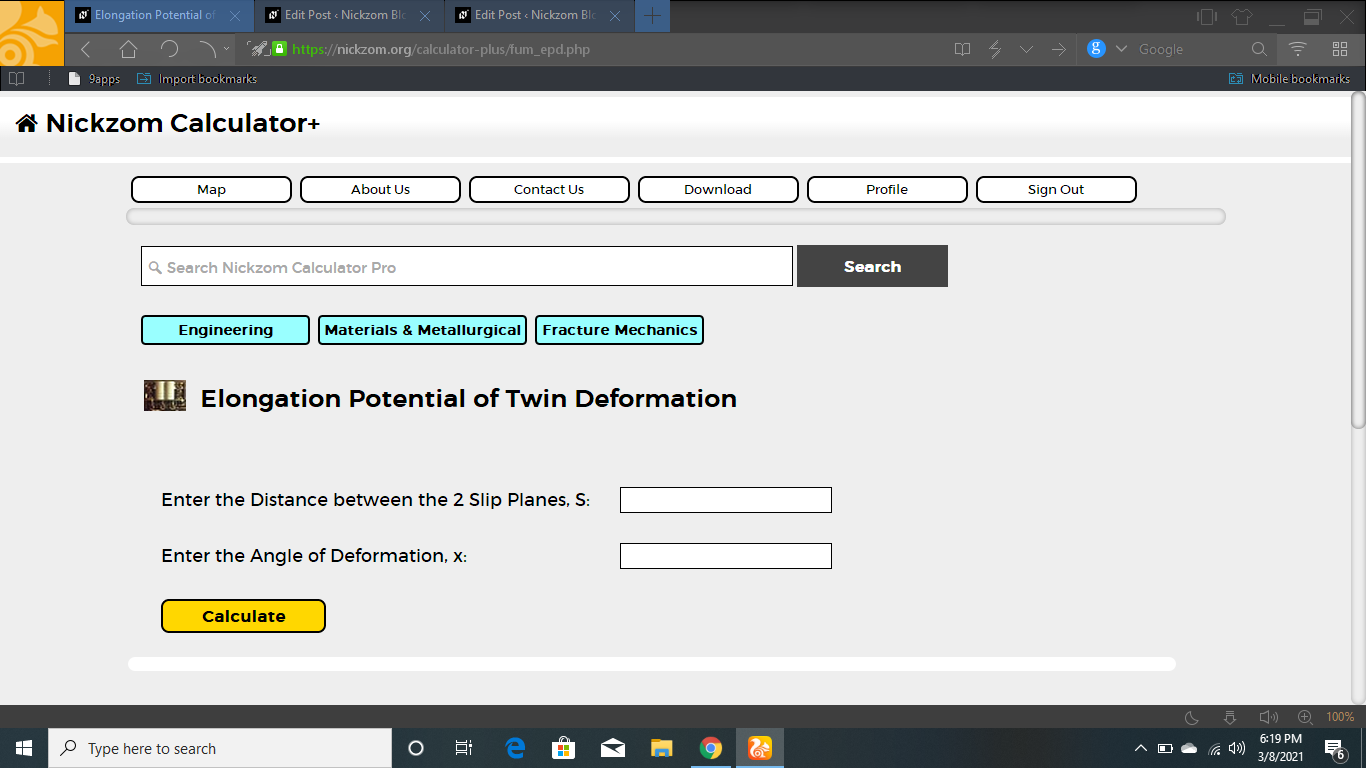Now, enter the value appropriately and accordingly for the parameter as required by the Distance between the 2 Slip Planes (S) is 24 and Angle of Deformation (x) is 12.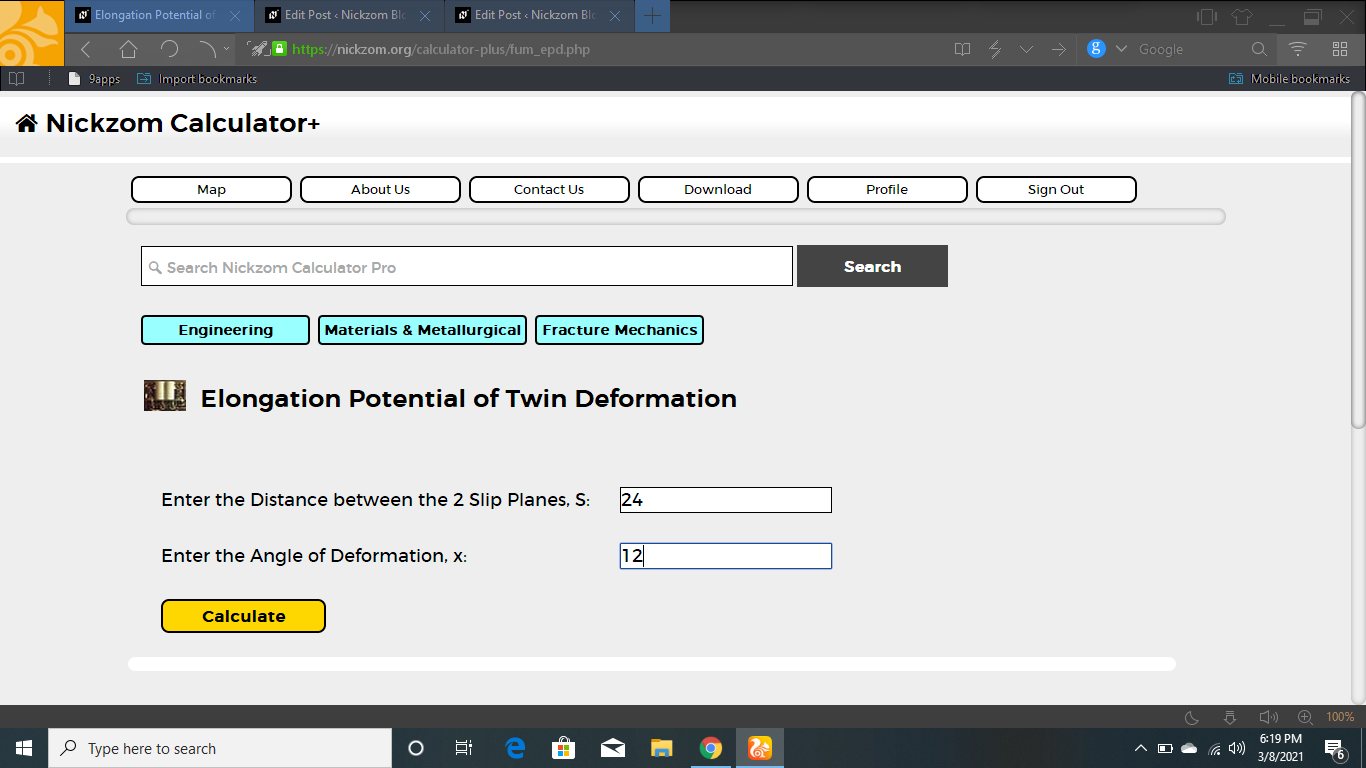Finally, Click on Calculate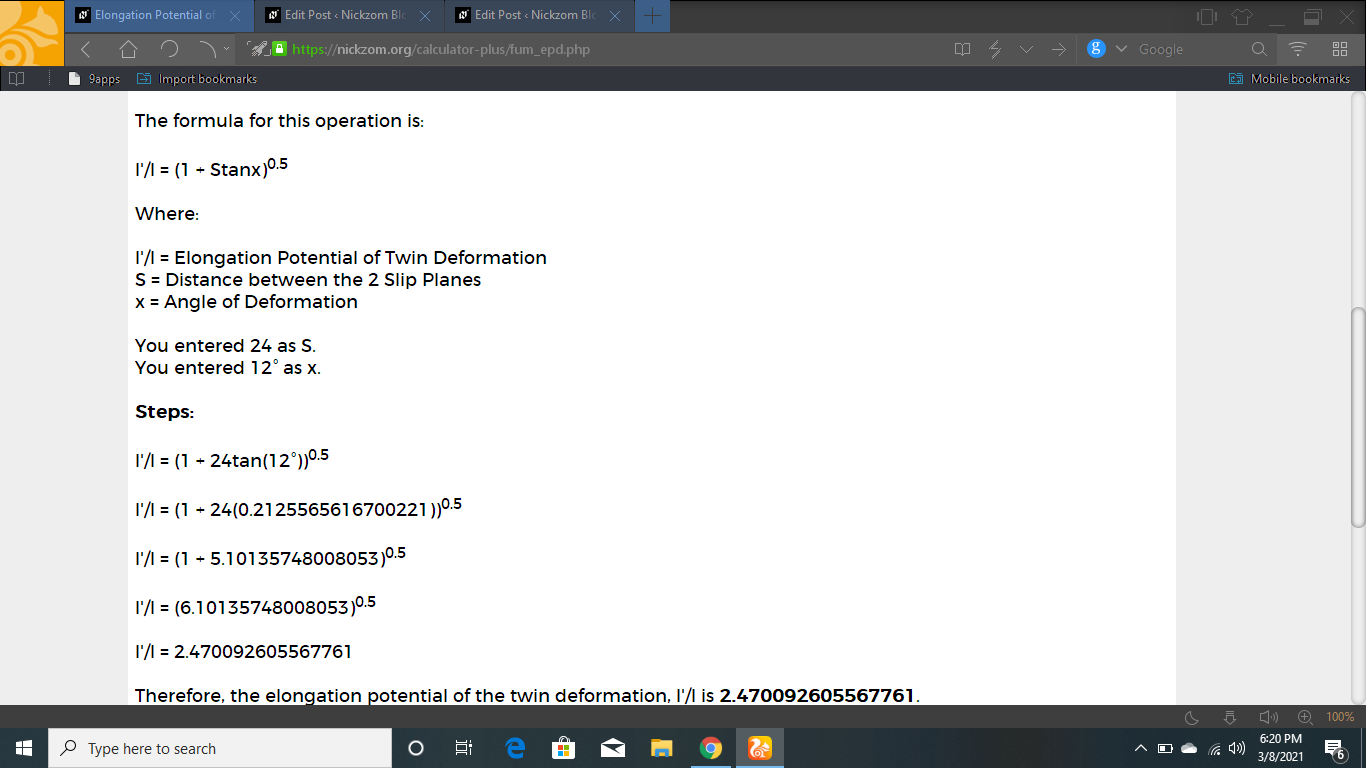As you can see from the screenshot above, Nickzom Calculator– The Calculator Encyclopedia solves for the elongation potential of twin deformation and presents the formula, workings and steps too.